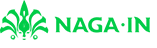@devilogic 2017-07-22T10:10:52.000000Z 字数 2331 阅读 1272

# 玩命的销售日志 2017.7.21

devilogic 日志

##• 状态集合 $S$ 描述了这个模型中存在的多少个状态。
• 转移函数 $\pi()$ 用于判断从上一个状态转移到哪个状态
• 状态的目标转移矩阵 $T$ 描述了转移矩阵的目标值，上一个状态的输出与此目标值越接近则选定哪个转移状态
• 当前状态的输入 $x_{ij}$ 表示第$i$个状态的第$j$个输入元素
• 当前状态的权值 $w_{ij}$ 表示第$i$个状态的第$j$个输入元素的权值
• 映射函数 $f()$ 表示单个节点输出的挤压函数，将节点的输出更加平滑，这里选取$sigmod$函数
• 当前状态输出 $o_{i}$$i$个状态的输出值

## 状态的目标转移矩阵

$\begin{bmatrix}t_{11}&t_{12}&t_{13}&\cdots&t_{1n}\\t_{21}&t_{22}&t_{23}&\cdots&t_{2n}\\\vdots&\vdots&\vdots&\ddots&\vdots\\t_{n1}&t_{n2}&t_{n3}&\cdots&t_{nn}\end{bmatrix}$

## 单状态的输出

$c$为倾斜参数（其变化会影响sigmoid函数的形状）。sigmoid函数的特点如下：对应值区间$(0,1)$，函数呈非线性递归光滑变化，但当输入$u \rightarrow \pm \infty$时，函数一致逼近于幅值$(0, 1)$。该函数是全局可微的。

## 选定下一个状态

$\pi()$中会依次遍历目的节点，并选取其中误差最小的节点作为目的节点。

## 一些还没考虑清楚的问题

1. 由于单个状态的输出只是计算了一个线性组合，最后用挤压函数映射到$(0,1)$范围内，这个线性组合其实逼近能力很有限，可能会造成与目标值误差过大。
2. 在修订权值时，由于是考虑上一个状态对当前状态的修订，并且忽略了当前层输入对输出的影响。这种修订完全没有考虑当前状态节点输入与上一个状态节点输入之间的联系。而如果这两种输入之间没有任何联系，那么这种修订则失去了意义。如何让上一个状态影响下一个状态并且这个影响也是同样与下一个状态的输入有所关联是这个问题的关键。
3. 目标值的设定，如何设定转移矩阵的目的目标值可以平均分布到输入状态权值向量张成的空间内。并保证这个设定也是容易的。
4. 权值初始值的设定，这要考虑到目标值的选取以及输入样本空间的问题。
5. 貌似还有很多。。。

## 小结• 私有
• 公开
• 删除